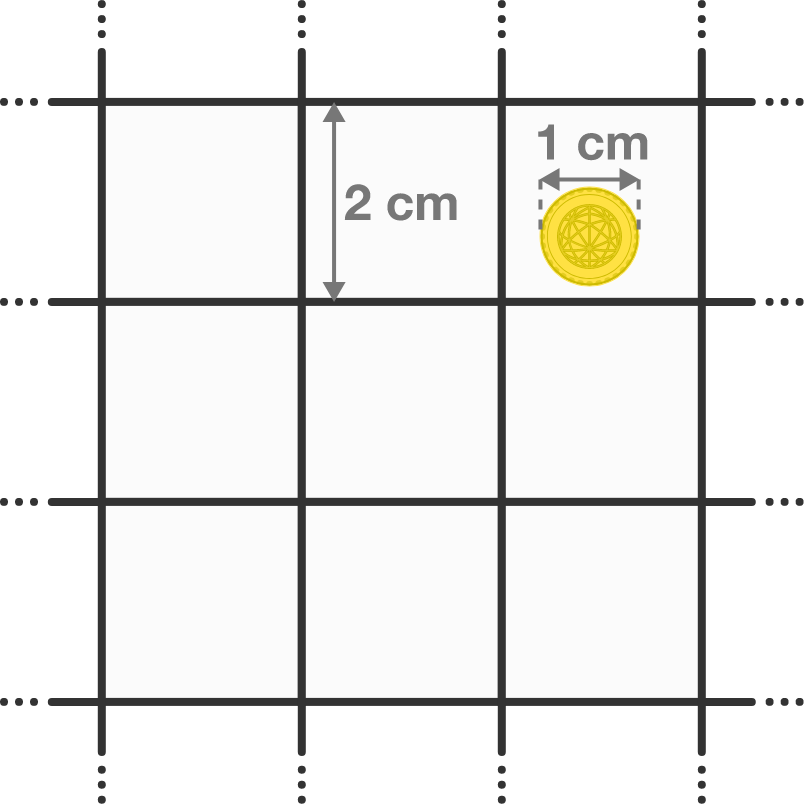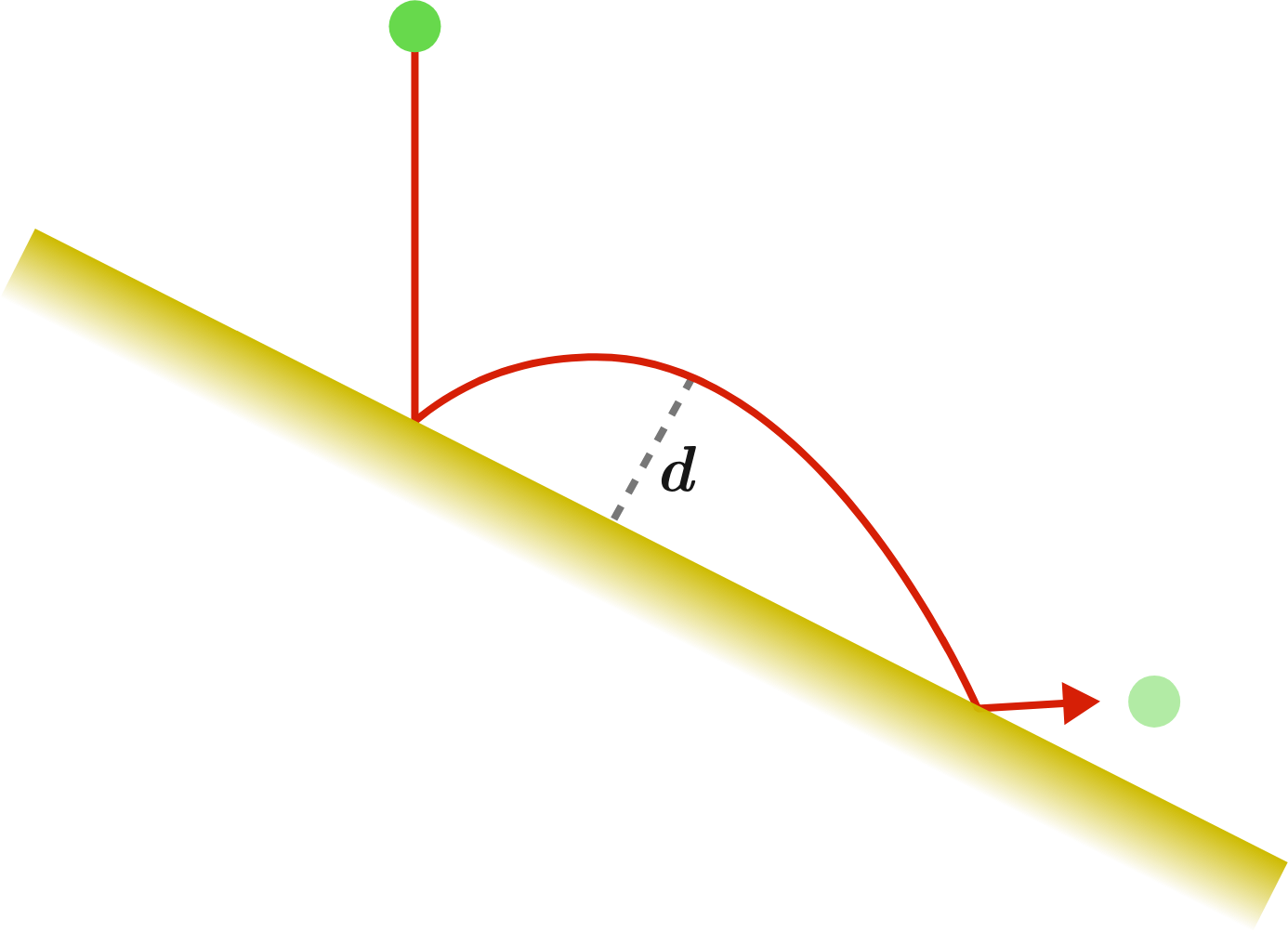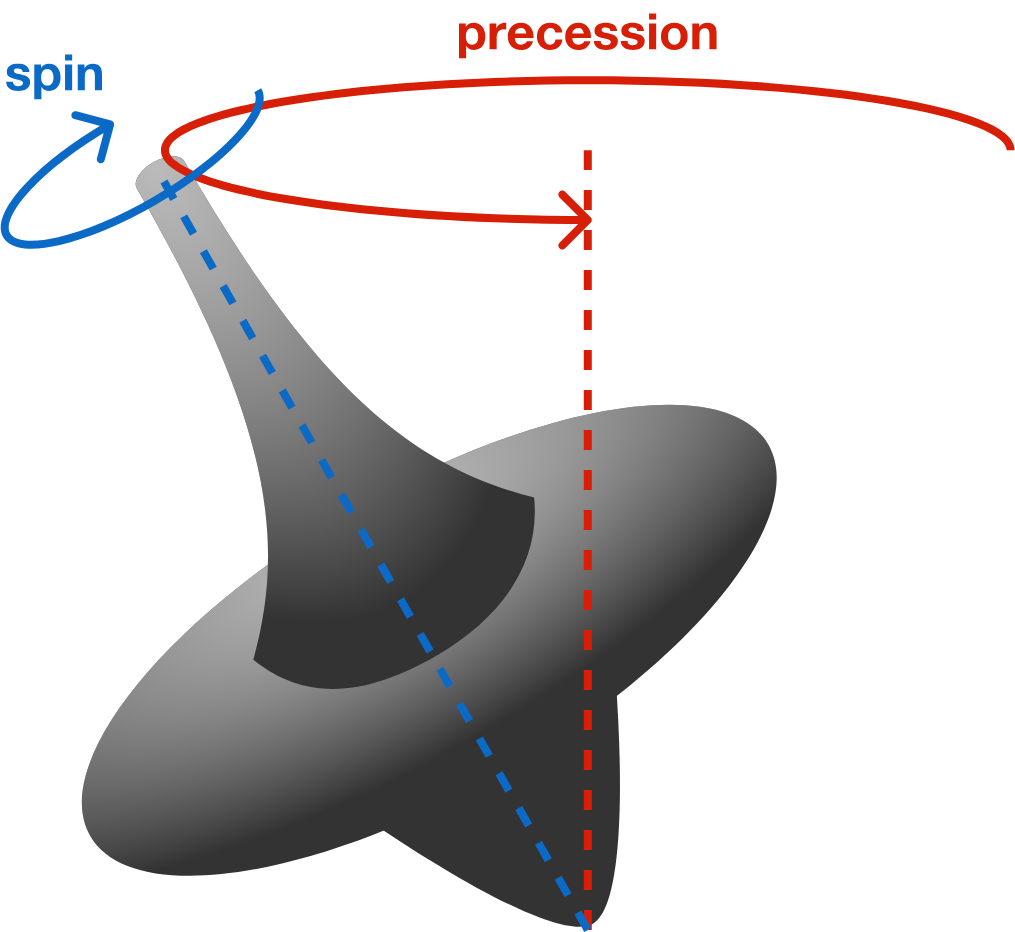# Problems of the Week

Contribute a problem

# 2018-10-01 Intermediate

A coin is randomly thrown on a square grid. As the size of the grid approaches infinity, what is the probability that the coin does not touch the gridlines?

Assume that the thickness of the grid lines is negligible.A ball is dropped perpendicular to the ground onto a slope.

How does the maximum distance $d$ from the ball to the slope vary as the ball rolls down?Details and Assumptions:

• Ignore air resistance.
• All collisions are perfectly elastic.A spinning top rotates about the axis passing through it as well as about a vertical axis. This motion is called precession.

The top's frequency of spinning decreases with time.

What happens to the top's frequency of precession with time?$a,b,c$ are integers satisfying

$a+b+c=a^2 + b^2+ c^2 =a^3 + b^3 + c^3.$

Are 0 and 1 the only possible values of $a,b,c?$

There are 8 players in a tennis tournament. At the beginning of the tournament, the players are matched at random. For each subsequent round, the losers are eliminated and the remaining players are matched at random.

The probability the $4^\text{th}$-best player makes it to the final match is $\frac{a}{b},$ where $a$ and $b$ are coprime positive integers.

What is $a+b?$

Assume that each player is distinctly skilled and that the better player always wins.

×